Posts in category optim

# Quaternion from two vectors: the final version

I have published a first article about how to build a quaternion from two arbitrary direction vectors that will transform one of the directions into the other. That article was deliberately omitting the special case when the two vectors were facing away from each other, which required special treatment. So I wrote a second article explaining how to quickly pick an orthogonal vector.

Combining the results from the two articles should be straightforward. Here is the resulting, final function:

/* Build a unit quaternion representing the rotation
* from u to v. The input vectors need not be normalised. */
quat quat::fromtwovectors(vec3 u, vec3 v)
{
float norm_u_norm_v = sqrt(dot(u, u) * dot(v, v));
float real_part = norm_u_norm_v + dot(u, v);
vec3 w;

if (real_part < 1.e-6f * norm_u_norm_v)
{
/* If u and v are exactly opposite, rotate 180 degrees
* around an arbitrary orthogonal axis. Axis normalisation
* can happen later, when we normalise the quaternion. */
real_part = 0.0f;
w = abs(u.x) > abs(u.z) ? vec3(-u.y, u.x, 0.f)
: vec3(0.f, -u.z, u.y);
}
else
{
/* Otherwise, build quaternion the standard way. */
w = cross(u, v);
}

return normalize(quat(real_part, w.x, w.y, w.z));
}


If you know you are exclusively dealing with unit vectors, you can replace all occurrences of norm_u_norm_v with the value 1.0f in order to avoid a useless square root.

# On picking an orthogonal vector (and combing coconuts)

Consider the following problem:

Given a non-zero vector v in 3D space, find a non-zero vector w orthogonal to v (i.e. such that v.w = 0).

At first sight, this seems easy. For every possible v there are an infinite number of orthogonal vectors lying in a plane:We just need to pick one. But it’s not as trivial as it may seem.

## First attempts

The following vector satisfies v.w1 = 0:Unfortunately that w1 vector can be zero, for instance when v = [0 0 1]. The original problem requires a non-zero vector.

Let’s try another one: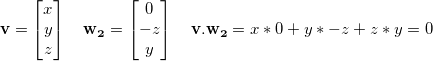Again, it works most of the time, except in some cases, for instance v = [1 0 0].

How about adding them together? Certainly w1 and w2 cannot be both zero at the same time!Alas, this still doesn’t work all the time: it fails with v = ![1 0 1].

Should we try harder? Isn’t there a linear combination of these that will work all the time?

Well, no. There isn’t.

## You can’t comb a coconut

Sadly, the hairy ball theorem, a consequence of Brouwer’s fixed-point theorem, states that any continuous function f that maps the set of unit vectors to orthogonal vectors takes the value zero somewhere.

Whichever way you look at the sphere of unit vectors, a continuous tangent field will always have a zero point:Most of the maths operations we usually do (adding, multiplying, taking square roots, etc.) are continuous. Fortunately, we are not limited to them: we can use a simple if() construct to create a discontinuity that will save us.

## A very good implementation

Here is my personal implementation of the orthogonal vector problem. It is very good. It has excellent numerical stability. It only does one test. It is short. It is beautiful. I really hope you consider using it.

/* Always works if the input is non-zero.
* Doesn’t require the input to be normalised.
* Doesn’t normalise the output. */
vec3 orthogonal(vec3 v)
{
return abs(v.x) > abs(v.z) ? vec3(-v.y, v.x, 0.0)
: vec3(0.0, -v.z, v.y);
}


Many implementations, such as Ogre3D’s, have additional tests or operations to perform this task, and use epsilons and normalised vectors to avoid singularities.

But our only test is actually enough: if |x|>|z|, it means the largest component in absolute value in v is either x or y. So we build a vector using x and y. Otherwise, the largest component in absolute value is either y or z. So we build a vector using y and z. That way, we ensure that the length of our returned vector never, ever comes near zero.

Whether some given code will cause inefficient branching is often unclear. In our case, it is very likely that the ternary operator will actually be branchless, with some help of the compiler and the hardware.

That said, how about we try to get rid of the ternary operator, just for fun?

## Going branchless for fun

Let’s see. We had these two candidate vectors, w1 and w2, which worked almost always except when specific values of v caused them to be zero. And whatever the constant k we may pick, a vector of the form w1 + k w2 will eventually take the value zero for some value of v, too, because of the hairy ball theorem.

Now here comes the trick. Instead of using a constant k, we use a function f(x,y,z). This is what our w vector looks like: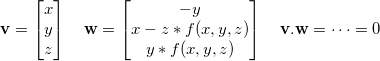From now I shall assume that v is a unit vector.

What can cause w to be zero, then?

One necessary condition is y = 0. When y ≠ 0 we can chose anything we want for f(x,y,z), it’ll always give us a good orthogonal vector. This restricts the problem to the y = 0 circle, giving us the useful equality x² + z² = 1.

The other condition is x = z*f(x,y,z). So if we manage to build a function f such that f(x,y,z) never equals x/z, we win.

Using x² + z² = 1 we can plot all the possible values for x/z as a function of x. It will show us, for a given x, the values that f cannot take:The almost vertical slopes you see go to infinity upwards and downwards. As expected, this prevents us from using a continuous function: it would obviously cross one of these walls at some point.

Well, let’s try a non-continuous function, then. What are our options?

• fmod
• floor, ceil, round
• fract

Here is one that I came up with and which works reasonably well: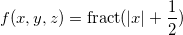Look how it nicely avoids x/z:And finally, our resulting branchless code:

/* Requires the input to be normalised.
* Doesn’t normalise the output. */
vec3 orthogonal(vec3 v)
{
float k = fract(abs(v.x) + 0.5);
return vec3(-v.y, v.x - k * v.z, k * v.y);
}


I find it highly unlikely that this second version will perform better than the branching one. However, I haven’t put much thought into it and maybe someone will come up with a much better solution using the ideas presented here. Have fun!

• Posted: 2013-09-21 11:25 (Updated: 2013-09-21 11:34)
• Author: sam
• Categories: maths optim

# Beautiful maths simplification: quaternion from two vectors

In this article I would like to explain the thought process behind the derivation of a widely used formula: finding a quaternion representing the rotation between two 3D vectors. Nothing really new, but hopefully a few ideas could be reused at other times.

Note: the routine presented here is incomplete on purpose. For a version that can be used in production code, see the next article instead, Quaternion from two vectors: the final version.

## Naive method

A rotation is best visualised using a rotation axis and an angle. Except in degenerate cases, the rotation axis can be obtained by computing the cross product of the two original vectors:Then the angle can be obtained using the properties of the cross product and/or the dot product:Since θ is always between 0 and π, we only care about the dot product. This gives us some obvious code to create the quaternion (omitting corner cases such as θ = 0 for clarity):

quat quat::fromtwovectors(vec3 u, vec3 v)
{
float cos_theta = dot(normalize(u), normalize(v));
float angle = acos(cos_theta);
vec3 w = normalize(cross(u, v));
return quat::fromaxisangle(angle, w);
}


This is naive but it works. Googling for “quaternion from two vectors” shows this forum post and this SO question where this method or a variation thereof is suggested.

## Looking under the hood

Let’s have a look at what happens when building the quaternion from an axis and an angle: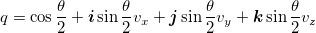This means the code for quat::fromaxisangle would look somewhat like this:

quat quat::fromaxisangle(float angle, vec3 axis)
{
float half_sin = sin(0.5f * angle);
float half_cos = cos(0.5f * angle);
return quat(half_cos,
half_sin * axis.x,
half_sin * axis.y,
half_sin * axis.z);
}


## Avoiding trigonometry

If you read Iñigo Quilez’s recent article about avoiding trigonometry you’ll have probably frowned at the fact that we computed θ from cos(θ), then computed sin(θ/2) and cos(θ/2).

Indeed, it happens that there is a much simpler way to do it; the half-angle formulas from precalculus tell us the following: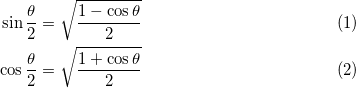This allows us to simplify our quaternion creation code:

quat quat::fromtwovectors(vec3 u, vec3 v)
{
float cos_theta = dot(normalize(u), normalize(v));
float half_cos = sqrt(0.5f * (1.f + cos_theta));
float half_sin = sqrt(0.5f * (1.f - cos_theta));
vec3 w = normalize(cross(u, v));
return quat(half_cos,
half_sin * w.x,
half_sin * w.y,
half_sin * w.z);
}


This is pretty nice. By using well known trigonometry formulas, we got rid of all trigonometry function calls!

## Avoiding square roots

It happens that we can do slightly better. Note that we normalize three vectors: u, v and cross(u, v). That’s three square roots. The thing is, we already know the norm of w through this formula: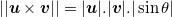And we know sin(θ) from precalculus again: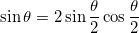Also, using the fact that sqrt(a)sqrt(b) = sqrt(ab) lets us perform one less square root.

We can therefore come up with the following performance improvement:

quat quat::fromtwovectors(vec3 u, vec3 v)
{
float norm_u_norm_v = sqrt(sqlength(u) * sqlength(v));
float cos_theta = dot(u, v) / norm_u_norm_v;
float half_cos = sqrt(0.5f * (1.f + cos_theta));
float half_sin = sqrt(0.5f * (1.f - cos_theta));
vec3 w = cross(u, v) / (norm_u_norm_v * 2.f * half_sin * half_cos);
return quat(half_cos,
half_sin * w.x,
half_sin * w.y,
half_sin * w.z);
}


Oh wait! We divide by sin(θ/2) to compute w, then we multiply by sin(θ/2) again. This means we don’t even need that variable, and we can simplify even further:

quat quat::fromtwovectors(vec3 u, vec3 v)
{
float norm_u_norm_v = sqrt(sqlength(u) * sqlength(v));
float cos_theta = dot(u, v) / norm_u_norm_v;
float half_cos = sqrt(0.5f * (1.f + cos_theta));
vec3 w = cross(u, v) / (norm_u_norm_v * 2.f * half_cos);
return quat(half_cos, w.x, w.y, w.z);
}


This is more or less the code used by the Ogre3D engine in OgreVector3.h, except they perform an additional normalisation step on the final result. This is mathematically useless, but due to numerical stability issues, it is probably safe to do so nonetheless.

This final normalisation step is actually an opportunity to simplify the code even further.

## Improving on Ogre3D

We are down to two square roots and four divisions, plus quite a few mul/adds. Depending on the platform that we are running on, it is possible to simplify even further and improve performance. For instance, on many SIMD architectures, normalising a quaternion can be very fast.

This is the code we get if we multiply every component of the quaternion by 2.f * half_cos and let normalize() do the rest of the job:

quat quat::fromtwovectors(vec3 u, vec3 v)
{
float norm_u_norm_v = sqrt(sqlength(u) * sqlength(v));
float cos_theta = dot(u, v) / norm_u_norm_v;
float half_cos = sqrt(0.5f * (1.f + cos_theta));
vec3 w = cross(u, v) / norm_u_norm_v;
return normalize(quat(2.f * half_cos * half_cos, w.x, w.y, w.z));
}


Now half_cos only appears in its squared form, and since it comes from a square root, we can simply omit that square root:

quat quat::fromtwovectors(vec3 u, vec3 v)
{
float norm_u_norm_v = sqrt(sqlength(u) * sqlength(v));
float cos_theta = dot(u, v) / norm_u_norm_v;
vec3 w = cross(u, v) / norm_u_norm_v;
return normalize(quat(1.f + cos_theta, w.x, w.y, w.z));
}


And using the same reasoning we can multiply every quaternion component by norm_u_norm_v:

quat quat::fromtwovectors(vec3 u, vec3 v)
{
float norm_u_norm_v = sqrt(sqlength(u) * sqlength(v));
vec3 w = cross(u, v);
quat q = quat(norm_u_norm_v + dot(u, v), w.x, w.y, w.z);
return normalize(q);
}


We are still doing two square roots and four divisions, some of which are hidden in normalize(), but the code is considerably shorter now.

## Final formIf u and v can be enforced to be unit vectors, norm_u_norm_v can be omitted and simply replaced with 1.0f:

quat quat::fromtwovectors(vec3 u, vec3 v)
{
vec3 w = cross(u, v);
quat q = quat(1.f + dot(u, v), w.x, w.y, w.z);
return normalize(q);
}


Isn’t it beautiful, considering the sin(), cos() and acos() ridden mess we started with?

This algorithm can be found all over the Internet, but I do not know who first came up with it. Also, a lot of 3D engines (both publicly available and slightly more private) could benefit from it.

## Update (06/01/2014)

In the comments below, Michael Norel provides the following improvement to the non-unit version. Since the values d = dot(u, v) and w = cross(u, v) are computed no matter what, the value sqlength(u) * sqlength(v) could be computed in a different way, *i.e.* d * d + sqlength(w). The following code does at least three multiplications less:

quat quat::fromtwovectors(vec3 u, vec3 v)
{
vec3 w = cross(u, v);
quat q = quat(dot(u, v), w.x, w.y, w.z);
q.w += length(q);
return normalize(q);
}


Also, Marc B. Reynolds notes that, in the unit version, the final normalisation factor is sqrt((1 + dot(u, v))² + sqlength(cross(u, v))) which reduces to sqrt(2 + 2 dot(u, v)) thanks to the sin² + cos² identity. It leads to the following possibly improved version:

quat quat::fromtwovectors(vec3 u, vec3 v)
{
float m = sqrt(2.f + 2.f * dot(u, v));
vec3 w = (1.f / m) * cross(u, v);
return quat(0.5f * m, w.x, w.y, w.z);
}

• Posted: 2013-09-18 18:13 (Updated: 2014-06-01 11:32)
• Author: sam
• Categories: maths optim

# Fast branchless RGB to HSV conversion in GLSL

Some time ago I devised an original algorithm to convert from RGB to HSV using very few CPU instructions and I wrote a small article about it.

When looking for a GLSL or HLSL conversion routine, I have found implementations of my own algorithm. However they were almost all straightforward, failing to take full advantage of the GPU’s advanced swizzling features.

So here it is, the best version I could come up with:

vec3 rgb2hsv(vec3 c)
{
vec4 K = vec4(0.0, -1.0 / 3.0, 2.0 / 3.0, -1.0);
vec4 p = mix(vec4(c.bg, K.wz), vec4(c.gb, K.xy), step(c.b, c.g));
vec4 q = mix(vec4(p.xyw, c.r), vec4(c.r, p.yzx), step(p.x, c.r));

float d = q.x - min(q.w, q.y);
float e = 1.0e-10;
return vec3(abs(q.z + (q.w - q.y) / (6.0 * d + e)), d / (q.x + e), q.x);
}


Update: Emil Persson suggests using the ternary operator explicitly to force compilers into using a fast conditional move instruction:

    vec4 p = c.g < c.b ? vec4(c.bg, K.wz) : vec4(c.gb, K.xy);
vec4 q = c.r < p.x ? vec4(p.xyw, c.r) : vec4(c.r, p.yzx);


And because a lot of people get it wrong, too, here is the reverse operation in GLSL. It is the algorithm almost everyone uses (or should use):

vec3 hsv2rgb(vec3 c)
{
vec4 K = vec4(1.0, 2.0 / 3.0, 1.0 / 3.0, 3.0);
vec3 p = abs(fract(c.xxx + K.xyz) * 6.0 - K.www);
return c.z * mix(K.xxx, clamp(p - K.xxx, 0.0, 1.0), c.y);
}


Porting to HLSL is straightforward: replace vec3 and vec4 with float3 and float4, mix with lerp, fract with frac, and clamp(…, 0.0, 1.0) with saturate(…).

• Posted: 2013-07-27 15:23 (Updated: 2013-07-28 11:54)
• Author: sam
• Categories: glsl optim

# A fast RGB to HSV floating point conversion

The operations typically performed to convert from RGB to HSV are the following:

• find the largest RGB component
• find the smallest RGB component
• compute V and S
• select the main circular sector for H
• compute H

Here is, to my knowledge, the most commonly used RGB to HSV routine for floating point, with an extra minor optimisation (adding 1e-20f to divisors to avoid the need to care about divisions by zero):

static void RGB2HSV(float r, float g, float b,
float &h, float &s, float &v)
{
float rgb_max = std::max(r, std::max(g, b));
float rgb_min = std::min(r, std::min(g, b));
float delta = rgb_max - rgb_min;
s = delta / (rgb_max + 1e-20f);
v = rgb_max;

float hue;
if (r == rgb_max)
hue = (g - b) / (delta + 1e-20f);
else if (g == rgb_max)
hue = 2 + (b - r) / (delta + 1e-20f);
else
hue = 4 + (r - g) / (delta + 1e-20f);
if (hue < 0)
hue += 6.f;
h = hue * (1.f / 6.f);
}


Several things seem worth noticing already:

• Most of the complexity comes from the hue calculation.
• Four min/max operations are performed to find rgb_max and rgb_min; however, sorting three values can be done with only 3 comparisons. This is not necessarily problematic because min/max could be wired in an efficient way depending on the CPU.
• Two additional tests are performed to compare r and g to rgb_max; if rgb_max and rgb_min were computed using tests, this is a waste of time to compare them again.
• Adding 6.f to the final hue value only has a 16.6% chance of happening.

The actual hue calculation depends on how r, g, and b are ordered: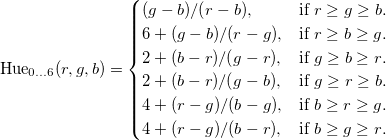But let’s rewrite this in terms of x, y and z, where x is the largest of (r,g,b), z is the smallest of the three, and y is inbetween: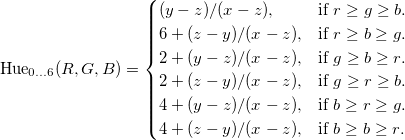There are a lot of similarities here. We can push it even further, using the fact that x ≥ z and y ≥ z by definition: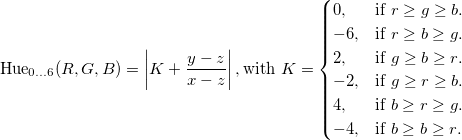That’s actually the same calculation! Only the hue offset K changes. The idea now is the following:

• Sort the triplet (r,g,b) using comparisons
• Build K while sorting the triplet
• Perform the final calculation

Putting the idea into practice gives us the following code:

static void RGB2HSV(float r, float g, float b,
float &h, float &s, float &v)
{
float K = 0.f;

if (g < b)
{
float tmp = g; g = b; b = tmp;
K = -1.f;
}

if (r < g)
{
float tmp = r; r = g; g = tmp;
K = -2.f / 6.f - K;
}

if (g < b)
{
float tmp = g; g = b; b = tmp;
K = -K;
}

float chroma = r - b;
h = fabs(K + (g - b) / (6.f * chroma + 1e-20f));
s = chroma / (r + 1e-20f);
v = r;
}


You can check for yourself that the values for K explicited above are properly generated by that function. There were many other ways to sort (r,g,b) but this specific one lets us do one final optimisation.

We notice that the last swap effectively changes the sign of K and the sign of g - b. Since both are then added and passed to fabs(), the sign reversal can actually be omitted.

That additional trickery gives us this final code:

static void RGB2HSV(float r, float g, float b,
float &h, float &s, float &v)
{
float K = 0.f;

if (g < b)
{
std::swap(g, b);
K = -1.f;
}

if (r < g)
{
std::swap(r, g);
K = -2.f / 6.f - K;
}

float chroma = r - std::min(g, b);
h = fabs(K + (g - b) / (6.f * chroma + 1e-20f));
s = chroma / (r + 1e-20f);
v = r;
}


That’s 2 tests and 1 std::min call instead of the previous 3 tests and 4 std::min/max calls. We really should see some kind of performance gain here.

And as expected, benchmarks indicate a performance increase of 25 to 40 % with a great variety of CPUs, compilers and compiler flags. The following graph (average nanoseconds per conversion) is on a Core i7-2600K CPU, using g++ 4.7.2 with -O3 -ffast-math:• Posted: 2013-01-13 06:19 (Updated: 2013-02-11 13:48)
• Author: sam
• Categories: optim c++

# Beyond De Bruijn: fast binary logarithm of a 10-bit number

Recently I needed a method for retrieving the binary logarithm of a 10-bit number (for the curious, it was for the purpose of converting between 32-bit and 16-bit floating point numbers).

Computing the binary logarithm is equivalent to knowing the position of the highest order set bit. For instance, log2(0x1) is 0 and log2(0x100) is 8.

One well known method for fast binary logarithm is presented at Bit Twiddling Hacks. It is a two-step method where first all lower bits are set to 1 and then a De Bruijn-like sequence is used to perform a table lookup:

int fastlog2(uint32_t v)
{
static const int MultiplyDeBruijnBitPosition =
{
0, 9, 1, 10, 13, 21, 2, 29, 11, 14, 16, 18, 22, 25, 3, 30,
8, 12, 20, 28, 15, 17, 24, 7, 19, 27, 23, 6, 26, 5, 4, 31
};

v |= v >> 1;
v |= v >> 2;
v |= v >> 4;
v |= v >> 8;
v |= v >> 16;

return MultiplyDeBruijnBitPosition[(uint32_t)(v * 0x07C4ACDDU) >> 27];
}


That is 12 integer operations and a table lookup.

## Optimising

It should be obvious what the sequence of operations on v does: fill the integer with ones starting from the highest order bit. Here are a few examples of what happens to v at each step:

 v v |= v >> 1 v |= v >> 2 v |= v >> 4 v |= v >> 8 v |= v >> 16 0x0001 0x0001 0x0001 0x0001 0x0001 0x0001 0x0002 0x0003 0x0003 0x0003 0x0003 0x0003 0x0003 0x0003 0x0003 0x0003 0x0003 0x0003 0x0004 0x0006 0x0007 0x0007 0x0007 0x0007 0x0100 0x0180 0x01e0 0x01fe 0x01ff 0x01ff 0x80000000 0xc0000000 0xf0000000 0xff000000 0xffff0000 0xffffffff

There is one obvious optimisation available: since the input is only 10-bit, the last shift operation v |= v >> 16 can be omitted because the final value was already reached.

int fastlog2(uint32_t v)
{
static const int MultiplyDeBruijnBitPosition =
{
0, 9, 1, 10, 13, 21, 2, 29, 11, 14, 16, 18, 22, 25, 3, 30,
8, 12, 20, 28, 15, 17, 24, 7, 19, 27, 23, 6, 26, 5, 4, 31
};

v |= v >> 1;
v |= v >> 2;
v |= v >> 4;
v |= v >> 8;

return MultiplyDeBruijnBitPosition[(uint32_t)(v * 0x07C4ACDDU) >> 27];
}


10 instructions instead of 12. Not really amazing, but worth mentioning.

## Optimising more?

Could we do better? Now the last line is v |= v >> 8; and it is only useful to propagate the 9th and 10th bits to positions 1 and 2. What happens if we omit that line? Let’s see:

• For most values of v, the expected value is obtained.
• For values of v with a highest order bit at 9th position, 0x1fe could be obtained instead of 0x1ff.
• For values of v with a highest order bit at 10th position, one of 0x3fc, 0x3fd or 0x3fe could be obtained instead of 0x3ff.

The list of possible output values would therefore be 0x1, 0x3, 0x7, 0xf, 0x1f, 0x3f, 0x7f, 0xff, 0x1fe, 0x1ff, 0x3fc, 0x3fd, 0x3fe, 0x3ff. What happens to these values when multiplying them with the De Bruijn sequence? Let's see:

 v v * 0x07C4ACDDU) >> 27 0x1 0 0x3 2 0x7 6 0xf 14 0x1f 30 0x3f 29 0x7f 27 0xff 23 0x1fe 15 0x1ff 16 0x3fc 30 0x3fd 31 0x3fe 0 0x3ff 1

Damn! Two values are colliding. It looks like we cannot omit the last line after all.

## Beyond De Bruijn

Let’s give another try at the problem. Usually De Bruijn sequences are built using either nontrivial algorithms, or brute force. Maybe we could find another sequence that has no collision? Or a sequence that is not a De Bruijn sequence but that works for our problem?

Well, let’s just brute force!

(2 seconds later)

int fastlog2(uint32_t v)
{
static const int MagicTable =
{
0, 1, 2, 8, -1, 3, 5, 9, 9, 7, 4, -1, 6, -1, -1, -1
};

v |= v >> 1;
v |= v >> 2;
v |= v >> 4;

return MagicTable[(uint32_t)(v * 0x5a1a1a2u) >> 28];
}


Down to 8 instructions instead of 12. And the lookup table is now half the size!

## Conclusion

It is possible for multiply-and-shift techniques similar to the De Bruijn sequence algorithm to exist for a larger set of problems. Brute forcing the search is a totally valid method for 32-bit multiplication.

# Maths trick: doing fewer comparisons

Note: this is not an optimisation. It is just one more tool you should have in your toolbox when looking for optimisations. It may be useful.

This is the trick: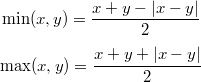You can check for yourself that it is always true: when x > y, |x - y| is the same as x - y, etc.

What good is it for? There is often an implicit comparison in min or max. It might be interesting to replace it with a call to the branchless fabs.

## Example usage

Consider the following code:

float a, b, c, d;
/* ... */
return (a > b) && (c > d);


That kind of code is often used eg. in collision checks, where a lot of tests can be done. This code does two comparisons. On some architectures, this means two branches. Not always something you want.

The test condition is equivalent to:

(a - b > 0) && (c - d > 0)


Now when are two given numbers both positive? That is if and only if the smallest is positive:

min(a - b, c - d) > 0


We may now use our trick:

(a - b) + (c - d) - |(a - b) - (c + d)| > 0


And so the code could be rewritten as such:

float a, b, c, d;
/* ... */
return (a - b) + (c - d) > fabsf((a - b) - (c - d));


We basically replaced the additional test with a call to fabsf and some additions/subtractions. It may be possible to reorganise the input data so that this second version performs better.

# Announce: LolRemez 0.2 released

A new version of our high precision polynomial approximation solver, LolRemez 0.2, is available.

The changes, taking into account all the feedback users provided, are as follows:

• significant performance and accuracy improvements thanks to various bugfixes and a better extrema finder for the error function.
• user can now define accuracy of the final result.
• exp, sin, cos and tan are now about 20% faster.
• multiplying a real number by an integer power of two is now a virtually free operation.
• fixed a rounding bug in the real number printing routine.

You can visit the software homepage to download LolRemez and, more importantly, the comprehensive documentation featuring a step-by-step tutorial.

# Announce: LolRemez 0.1 released

In my previous article about the Remez exchange algorithm I said I was working on a Remez exchange toolkit for everyone to play with. Though it’s far from being full-featured, I already use it and I believe it is already extremely useful. So I decided to release LolRemez 0.1 to the masses.

You can visit the software homepage to download LolRemez and, more importantly, the comprehensive documentation featuring a step-by-step tutorial.

# Better function approximations: Taylor vs. Remez

You may have once crossed this particular piece of magic: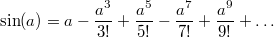The right part is the Taylor series of sin around 0. It converges very quickly to the actual value of sin(a). This allows a computer to compute the sine of a number with arbitrary precision.

And when I say it’s magic, it’s because it is! Some functions, called the entire functions, can be computed everywhere using one single formula! Other functions may require a different formula for different intervals; they are the analytic functions, a superset of the entire functions. In general, Taylor series are an extremely powerful tool to compute the value of a given function with very high accuracy, because for several common functions such as sin, tan or exp the terms of the series are easy to compute and, when implemented on a computer, can even be stored in a table at compile time.

## Approximating sin with Taylor series

This is how one would approximate sin using 7 terms of its Taylor series on the [-π/2, π/2] interval. The more terms, the better the precision, but we’ll stop at 7 for now:

static double taylorsin(double x)
{
static const
double a0 =  1.0,
a1 = -1.666666666666666666666666666666e-1,  /* -1/3! */
a2 =  8.333333333333333333333333333333e-3,  /*  1/5! */
a3 = -1.984126984126984126984126984126e-4,  /* -1/7! */
a4 =  2.755731922398589065255731922398e-6,  /*  1/9! */
a5 = -2.505210838544171877505210838544e-8,  /* -1/11! */
a6 =  1.605904383682161459939237717015e-10; /*  1/13! */
double x2 = x * x;
return x * (a0 + x2 * (a1 + x2 * (a2 + x2
* (a3 + x2 * (a4 + x2 * (a5 + x2 * a6))))));
}


And you may think…“Oh wow that is awesome! So simple for such a difficult function. Also, since I read your masterpiece about polynomial evaluation I know how to improve that function so that it is very fast!”

Well, actually, no.If you are approximating a function over an interval using its Taylor series then either you or the person who taught you is a fucking idiot because a Taylor series approximates a function near a fucking point, not over a fucking interval, and if you don’t understand why it’s important then please read on because that shit is gonna blow your mind.

## Error measurement

Let’s have a look at how much error our approximation introduces. The formula for the absolute error is simple: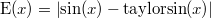And this is how it looks like over our interval:You can see that the error skyrockets near the edges of the [-π/2, π/2] interval.“Well the usual way to fix this is to split the interval in two or more parts, and use a different Taylor series for each interval.”

Oh, really? Well, let’s see the error on [-π/4, π/4] instead:I see no difference! The error is indeed smaller, but again, it becomes extremely large at the edges of the interval. And before you start suggesting reducing the interval even more, here is the error on [-π/8, π/8] now:I hope this makes it clear that:

• the further from the centre of the interval, the larger the error
• the error distribution is very unbalanced
• the maximum error on [-π/2, π/2] is about 6.63e-10

And now I am going to show you why that maximum error value is pathetic.

## A better approximation

Consider this new function:

static double minimaxsin(double x)
{
static const
double a0 =  1.0,
a1 = -1.666666666640169148537065260055e-1,
a2 =  8.333333316490113523036717102793e-3,
a3 = -1.984126600659171392655484413285e-4,
a4 =  2.755690114917374804474016589137e-6,
a5 = -2.502845227292692953118686710787e-8,
a6 =  1.538730635926417598443354215485e-10;
double x2 = x * x;
return x * (a0 + x2 * (a1 + x2 * (a2 + x2
* (a3 + x2 * (a4 + x2 * (a5 + x2 * a6))))));
}


It doesn’t look very different, right? Right. The values a0 to a6 are slightly different, but the rest of the code is strictly the same.

Yet what a difference it makes! Look at this error curve:That new function makes it obvious that:

• the error distribution is better spread over the interval
• the maximum error on [-π/2, π/2] is about 4.96e-14

Check that last figure again. The new maximum error isn’t 10% better, or maybe twice as good. It is more than ten thousand times smaller!!

## The minimax polynomial

The above coefficients describe a minimax polynomial: that is, the polynomial that minimises a given error when approximating a given function. I will not go into the mathematical details, but just remember this: if the function is sufficiently well-suited (as sin, tan, exp etc. are), then the minimax polynomial can be found.

The problem? It’s hard to find. The most popular algorithm to find it is the Remez exchange algorithm, and few people really seem to understand how it works (or there would be a lot less Taylor series). I am not going to explain it right now. Usually you need professional math tools such as Maple or Mathematica if you want to compute a minimax polynomial. The Boost library is a notable exception, though.

But you saw the results, so stop using Taylor series. Spending some time finding the minimax polynomial is definitely worth it. This is why I am working on a Remez framework that I will make public and free for everyone to use, modify and do what the fuck they want. In the meantime, if you have functions to numerically approximate, or Taylor-based implementations that you would like to improve, let me know in the comments! This will be great use cases for me.

# GLSL code snippet: choosing from 4 vectors by Z value

There aren’t many low-level GLSL optimisation resources out there, so I decided that I would share my thoughts when working on some specific parts of my code.

## The basic, complete shader function

This one time I had four vec3 vectors, with an xy texture coordinate, and a weight stored in z. The code to compute the final pixel value was:

vec4 getColor(vec3 a, vec3 b, vec3 c, vec3 d)
{
vec4 pa = texture2D(tex, a.xy) * a.z;
vec4 pb = texture2D(tex, b.xy) * b.z;
vec4 pc = texture2D(tex, c.xy) * c.z;
vec4 pd = texture2D(tex, d.xy) * d.z;
return (pa + pb + pc + pd) / (a.z + b.z + c.z + d.z);
}


That is four texture lookups, which is expensive.

## The lightweight version for coarse levels of detail

If I wanted a more lightweight fragment shader, for instance when implementing variable levels of shader complexity, I would want to do only one texture lookup, and use the vector with the largest weight:

vec4 getColorFast(vec3 a, vec3 b, vec3 c, vec3 d)
{
if (a.z < c.z) // These two tests are
a = c;     // likely to be run in
if (b.z < d.z) // parallel because they use
b = d;     // independent data.
if (a.z < b.z)
a = b;
return texture2D(tex, a.xy);
}


Only one texture lookup, but three branches. Branches are expensive and should be avoided.

Fortunately, GLSL provides step() and mix() (in HLSL or Cg, step() and lerp()) that let us do things similar to fsel on the PowerPC, or vec_sel in AltiVec: a branch-free select.

vec4 getColorFaster(vec3 a, vec3 b, vec3 c, vec3 d)
{
a = mix(a, c, step(a.z, c.z)); // Again, potentially good
b = mix(b, d, step(b.z, d.z)); // scheduling between these lines
a = mix(a, b, step(a.z, b.z));
return texture2D(tex, a.xy);
}


Excellent! Only six instructions in addition to the texture lookup.

But if you are familiar with SSE or AltiVec-style SIMD programming on the CPU, you will know this is not the usual way to do. Rather than 4 vectors of 3 elements, SIMD programming prefers to work in parallel on 3 vectors of 4 X, Y and Z components:

vec4 getColorShuffled(vec4 allx, vec4 ally, vec4 allz)
{
/* Now what do we do here? */
}


One nice thing to realise is that the equivalent of our previous step(a.z, c.z) and step(b.z, d.z) tests can be done in parallel:

vec4 getColorShuffled(vec4 allx, vec4 ally, vec4 allz)
{
// compare a.z >? c.z and b.z >? d.z in parallel
vec2 t = step(vec2(allz, allz), vec2(allz, allz));
// choose between a and c using t, between b and d using t
vec2 twox = mix(vec2(allx, allx), vec2(allx, allx), t);
vec2 twoy = mix(vec2(ally, ally), vec2(ally, ally), t);
vec2 twoz = mix(vec2(allz, allz), vec2(allz, allz), t);
// compare a.z and b.z
float s = step(twoz, twoz);
// now choose between a and b using s
vec2 best = vec2(mix(twox, twox, t2), mix(twoy, twoy, s));
return texture2D(tex, best);
}


Wow, that’s a bit complex. And even if we’re doing two calls to step() instead of three, there are now five calls to mix() instead of three. Fortunately, thanks to swizzling, we can combine most of these calls to mix():

vec4 getColorShuffledFast(vec4 allx, vec4 ally, vec4 allz)
{
vec2 t = step(allz.ag, allz.rb);
vec4 twoxy = mix(vec4(allx.ag, ally.ag), vec4(allx.rb, ally.rb), t.xyxy);
vec2 twoz  = mix(allz.ag, allz.rb, t);
float t2 = step(twoz.a, twoz.r);
vec2 best = mix(twoxy.ag, twoxy.rb, t2);
return texture2D(tex, best);
}


That’s it! Only three mix() and two step() instructions. Quite a few swizzles, but these are extremely cheap on modern GPUs.

## Afterthoughts

The above transformation was at the “cost” of a big data layout change known as array of structures to structure of arrays. When working in parallel on similar data, it is very often a good idea, and the GPU was no exception here.

This was actually a life saver when trying to get a fallback version of a shader to work on an i915 card, where mix and step must be emulated using ALU instructions, up to a maximum of 64. The result can be seen in this NaCl plugin.

• Posted: 2011-11-30 23:53 (Updated: 2011-12-04 11:53)
• Author: sam
• Categories: glsl optim

# Playing with the CPU pipeline

This article will show how basic knowledge of a modern CPU’s instruction pipeline can help micro-optimise code at very little cost, using a real world example: the approximation of a trigonometric function. All this without necessarily having to look at lines of assembly code.

## Evaluating polynomials

Who needs polynomials anyway? We’re writing games, not a computer algebra system, after all. But wait! Taylor series are an excellent mathematical tool for approximating certain classes of functions. For instance, this is the Taylor series of sin(x) near x = 0: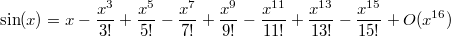Truncating the series at the 15th power will compute sin(x) with an absolute error no greater than 1e-11 in the range [-π/2; π/2], and 2e-16 in the range [-π/4; π/4].

However, a better approximation known as the minimax polynomial (probably featured in an upcoming article) will give a maximum absolute error of about 2e-16 on the whole [-π/2; π/2] range:

static double a0 = +1.0;
static double a1 = -1.666666666666580809419428987894207e-1;
static double a2 = +8.333333333262716094425037738346873e-3;
static double a3 = -1.984126982005911439283646346964929e-4;
static double a4 = +2.755731607338689220657382272783309e-6;
static double a5 = -2.505185130214293595900283001271652e-8;
static double a6 = +1.604729591825977403374012010065495e-10;
static double a7 = -7.364589573262279913270651228486670e-13;

double sin1(double x)
{
return a0 * x
+ a1 * x * x * x
+ a2 * x * x * x * x * x
+ a3 * x * x * x * x * x * x * x
+ a4 * x * x * x * x * x * x * x * x * x
+ a5 * x * x * x * x * x * x * x * x * x * x * x
+ a6 * x * x * x * x * x * x * x * x * x * x * x * x * x
+ a7 * x * x * x * x * x * x * x * x * x * x * x * x * x * x * x;
}


That is 64 multiplications and 7 additions, though compiler options such as GCC’s -ffast-math will help factor the expression in order to perform fewer operations.

It is possible to help the CPU by noticing that a term such as x^9 can be computed in only one operation if x^2 and x^7 are already known, leading to the following code:

double sin2(double x)
{
double ret, y = x, x2 = x * x;
ret = a0 * y; y *= x2;
ret += a1 * y; y *= x2;
ret += a2 * y; y *= x2;
ret += a3 * y; y *= x2;
ret += a4 * y; y *= x2;
ret += a5 * y; y *= x2;
ret += a6 * y; y *= x2;
ret += a7 * y;
return ret;
}


That is now only 16 multiplications and 7 additions. But it is possible to do even better using the Horner form of a polynomial evaluation: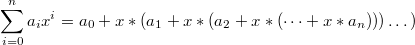double sin3(double x)
{
double x2 = x * x;
return x * (a0 + x2 * (a1 + x2 * (a2 + x2 * (a3 + x2 * (a4 + x2 * (a5 + x2 * (a6 + x2 * a7)))))));
}


We are down to 9 multiplications and 7 additions. There is probably no way to be faster, is there? Let’s see…

## Timings

Here are the timings in nanoseconds for the above code, compared with the glibc’s sin() function. The test CPU is an Intel® Core™ i7-2620M CPU at 2.70GHz. The functions were compiled using -O3 -ffast-math:

 function sin sin1 sin2 sin3 nanoseconds per call 22.518 16.406 16.658 25.276

Wait, what? Our superbly elegant function, performing only 9 multiplications, is actually slower than the 64-multiplication version? Which itself is as fast as the 16-multiplication one? Surely we overlooked something.

That’s right. We ignored the CPU pipeline.

## The instruction pipeline

In order to execute an instruction, such as “add A and B into C”, a CPU needs to do at least the following:

• fetch the instruction (ie. read it from the program’s memory)
• decode the instruction
• read the instruction’s operands (ie. A and B)
• execute the instruction
• write the result in memory or in registers (in our case, C)

On a modern Intel® CPU, the execution step only accounts for 1/10 or even 1/16 of the total execution time. The idea behind pipelining is simple: while executing an instruction, the CPU can often already read the operands for the next one.

But there is a problem with this strategy: if the next instruction depends on the result of the current one, the CPU cannot read the next operands yet. This is called a read-after-write hazard, and most usually causes a pipeline stall: the CPU just does nothing until it can carry on.

For the sake of simplicity, imagine the CPU’s pipeline depth is 3. At a given time, it can fetch, execute and finish one instruction:

 ● instruction is being fetched, executed or finished ○ instruction could start, but needs to wait for the result of a previous instruction

This is how the CPU would execute A = (a + b) * (c + d):

 time → total: 7 1 B = a + b ● ● ● 2 C = c + d ● ● ● 3 A = B * C ○ ○ ● ● ●

The c + d operation can be started very early because it does not depend on the result of a + b. This is called instruction-level parallelism. However, the final B * C operation needs to wait for all previous instructions to finish.

Since every operation in sin3() depends on the previous one, this is how it would execute that function:

 time → total: 48 1 x2 = x * x ● ● ● 2 A = a7 * x2 ○ ○ ● ● ● 3 A += a6 ○ ○ ● ● ● 4 A *= x2 ○ ○ ● ● ● 5 A += a5 ○ ○ ● ● ● 6 A *= x2 ○ ○ ● ● ● 7 A += a4 ○ ○ ● ● ● 8 A *= x2 ○ ○ ● ● ● 9 A += a3 ○ ○ ● ● ● 10 A *= x2 ○ ○ ● ● ● 11 A += a2 ○ ○ ● ● ● 12 A *= x2 ○ ○ ● ● ● 13 A += a1 ○ ○ ● ● ● 14 A *= x2 ○ ○ ● ● ● 15 A += a0 ○ ○ ● ● ● 16 A *= x ○ ○ ● ● ●

These 9 multiplications and 7 additions are done in 48 units of time. No instruction-level parallelism is possible because each instruction needs to wait for the previous one to finish.

The secret behind sin2()’s performance is that the large number of independent operations allows the compiler to reorganise the computation so that the instructions can be scheduled in a much more efficient way. This is roughly how GCC compiled it:

 time → total: 30 1 x2 = x * x ● ● ● 2 A = a7 * x2 ○ ○ ● ● ● 3 x3 = x2 * x ● ● ● 4 A += a6 ○ ● ● ● 5 B = a1 * x3 ● ● ● 6 x5 = x3 * x2 ● ● ● 7 A *= x2 ● ● ● 8 C = a2 * x5 ○ ● ● ● 9 B += x ● ● ● 10 x7 = x5 * x2 ● ● ● 11 A += a5 ● ● ● 12 D = a3 * x7 ● ● ● 13 B += C ● ● ● 14 x9 = x7 * x2 ● ● ● 15 B += D ○ ● ● ● 16 E = a4 * x9 ● ● ● 17 x11 = x9 * x2 ● ● ● 18 B += E ○ ● ● ● 19 A *= x11 ● ● ● 20 A += B ○ ○ ● ● ●

These 13 multiplications and 7 additions are executed in 30 units of time instead of 48 for the previous version. The compiler has been rather clever here: the number of ○’s is kept small.

Note that 30 / 48 = 0.625, and the ratio between sin2 and sin3’s timings is 16.658 / 25.276 = 0.659. Reality matches theory pretty well!

## Going further

We have seen that increasing the number of operations in order to break dependencies between CPU instructions allowed to help the compiler perform better optimisations taking advantage of the CPU pipeline. But that was at the cost of 40% more multiplications. Maybe there is a way to improve the scheduling without adding so many instructions?

Luckily there are other ways to evaluate a polynomial.

## Even-Odd form and similar schemes

Consider our 8th order polynomial: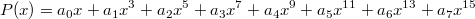Separating the odd and even coefficients, it can be rewritten as: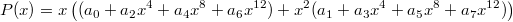Which using Horner’s form yields to: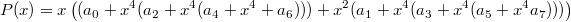This polynomial evaluation scheme is called the Even-Odd scheme. It only has 9 multiplications and 7 additions (only one multiplication more than the optimal case). It results in the following C code:

double sin4(double x)
{
double x2 = x * x;
double x4 = x2 * x2;
double A = a0 + x4 * (a2 + x4 * (a4 + x4 * a6));
double B = a1 + x4 * (a3 + x4 * (a5 + x4 * a7));
return x * (A + x2 * B);
}


And this is the expected scheduling:

 time → total: 33 1 x2 = x * x ● ● ● 2 x4 = x2 * x2 ○ ○ ● ● ● 3 B = a7 * x4 ○ ○ ● ● ● 4 A = a6 * x4 ● ● ● 5 B += a5 ○ ● ● ● 6 A += a4 ● ● ● 7 B *= x4 ○ ● ● ● 8 A *= x4 ● ● ● 9 B += a3 ○ ● ● ● 10 A += a2 ● ● ● 11 B *= x4 ○ ● ● ● 12 A *= x4 ● ● ● 13 B += a1 ○ ● ● ● 14 A += a0 ● ● ● 15 B *= x2 ○ ● ● ● 16 A += B ○ ○ ● ● ● 17 A *= x ○ ○ ● ● ●

Still not good enough, but we’re certainly onto something here. Let’s try another decomposition for the polynomial: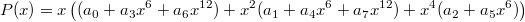And using Horner’s form again: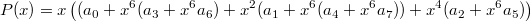Resulting in the following code:

double sin5(double x)
{
double x2 = x * x;
double x4 = x2 * x2;
double x6 = x4 * x2;
double A = a0 + x6 * (a3 + x6 * a6);
double B = a1 + x6 * (a4 + x6 * a7);
double C = a2 + x6 * a5;
return x * (A + x2 * B + x4 * C);
}


And the following scheduling:

 time → total: 31 1 x2 = x * x ● ● ● 2 x4 = x2 * x2 ○ ○ ● ● ● 3 x6 = x4 * x2 ○ ○ ● ● ● 4 B = x6 * a7 ○ ○ ● ● ● 5 A = x6 * a6 ● ● ● 6 C = x6 * a5 ● ● ● 7 B += a4 ● ● ● 8 A += a3 ● ● ● 9 C += a2 ● ● ● 10 B *= x6 ● ● ● 11 A *= x6 ● ● ● 12 C *= x4 ● ● ● 13 B += a1 ● ● ● 14 A += a0 ● ● ● 15 B *= x2 ○ ● ● ● 16 A += C ● ● ● 17 A += B ○ ○ ● ● ● 18 A *= x ○ ○ ● ● ●

One more instruction and two units of time better. That’s slightly better, but still not as good as we would like. One problem is that a lot of time is lost waiting for the value x6 to be ready. We need to find computations to do in the meantime to avoid pipeline stalls.

## High-Low form

Instead of splitting the polynomial into its even and odd coefficients, we split it into its high and low coefficients:And again using Horner’s form:The corresponding code is now:

double sin6(double x)
{
double x2 = x * x;
double x4 = x2 * x2;
double x8 = x4 * x4;
double A = a0 + x2 * (a1 + x2 * (a2 + x2 * a3));
double B = a4 + x2 * (a5 + x2 * (a6 + x2 * a7));
return x * (A + x8 * B);
}


And the expected scheduling:

 time → total: 30 1 x2 = x * x ● ● ● 2 B = x2 * a7 ○ ○ ● ● ● 3 A = x2 * a3 ● ● ● 4 x4 = x2 * x2 ● ● ● 5 B += a6 ● ● ● 6 A += a2 ● ● ● 7 x8 = x4 * x4 ● ● ● 8 B *= x2 ● ● ● 9 A *= x2 ● ● ● 10 B += a5 ○ ● ● ● 11 A += a1 ● ● ● 12 B *= x2 ○ ● ● ● 13 A *= x2 ● ● ● 14 B += a4 ○ ● ● ● 15 A += a0 ● ● ● 16 B *= x8 ○ ● ● ● 17 A += B ○ ○ ● ● ● 18 A *= x ○ ○ ● ● ●

Finally! We now schedule as well as GCC, and with 11 multiplications instead of 13. Still no real performance gain, though.

## Pushing the limits

Can we do better? Probably. Remember that each ○ in the above table is a pipeline stall, and any instruction we would insert there would be basically free.

Note the last instruction, A *= x. It causes a stall because it needs to wait for the final value of A, but it would not be necessary if A and B had been multiplied by x beforehands.

Here is a way to do it (bold instructions indicate a new instruction or a modified one):

 time → total: 27 1 x2 = x * x ● ● ● 2 B = x2 * a7 ○ ○ ● ● ● 3 A = x2 * a3 ● ● ● 4 x4 = x2 * x2 ● ● ● 5 B += a6 ● ● ● 6 A += a2 ● ● ● 7 x8 = x4 * x4 ● ● ● 8 B *= x2 ● ● ● 9 A *= x2 ● ● ● 10 x3 = x2 * x ● ● ● 11 B += a5 ● ● ● 12 A += a1 ● ● ● 13 C = a0 * x ● ● ● 14 B *= x2 ● ● ● 15 A *= x3 ● ● ● 16 x9 = x8 * x ● ● ● 17 B += a4 ● ● ● 18 A += C ● ● ● 19 B *= x9 ○ ● ● ● 20 A += B ○ ○ ● ● ●

Excellent! Just as many instructions as GCC, but now with fewer pipeline stalls. I don’t know whether this scheduling is optimal for the (incorrect) assumption of a 3-stage pipeline, but it does look pretty good. Also, loading a0, a1 etc. from memory hasn't been covered for the sake of simplicity.

Anyway, we just need to write the code corresponding to this behaviour, and hope the compiler understands what we need:

double sin7(double x)
{
double x2 = x * x;
double x3 = x2 * x;
double x4 = x2 * x2;
double x8 = x4 * x4;
double x9 = x8 * x;
double A = x3 * (a1 + x2 * (a2 + x2 * a3));
double B = a4 + x2 * (a5 + x2 * (a6 + x2 * a7));
double C = a0 * x;
return A + C + x9 * B;
}


## Conclusion

It’s time to check the results! Here they are, for all the functions covered in this article:

 function sin sin1 sin2 sin3 sin4 sin5 sin6 sin7 nanoseconds per call 22.518 16.406 16.658 25.276 18.666 18.582 16.366 17.470

Damn. All these efforts to understand and refactor a function, and our best effort actually performs amongst the worst!

What did we miss? Actually, this time, nothing. The problem is that GCC didn't understand what we were trying to say in sin7() and proceeded with its own optimisation ideas. Compiling with -O3 instead of -O3 -ffast-math gives a totally different set of timings:

 function sin sin1 sin2 sin3 sin4 sin5 sin6 sin7 nanoseconds per call 22.497 30.250 19.865 25.279 18.587 18.958 16.362 15.891

There. We win eventually!

There is a way to still use -ffast-math yet prevent GCC from trying to be too clever. This might be preferable because we do not want to lose the benefits of -ffast-math in other places. By using an architecture-specific assembly construct, we can mark temporary variables as used, effectively telling GCC that the variable needs to be really computed and not optimised away:

double sin7(double x)
{
double x2 = x * x;
double x3 = x2 * x;
double x4 = x2 * x2;
double x8 = x4 * x4;
double x9 = x8 * x;
#if defined __x86_64__
__asm__("" : "+x" (x3), "+x" (x9));
#elif defined __powerpc__ || defined __powerpc64__
__asm__("" : "+f" (x3), "+f" (x9));
#else
__asm__("" : "+m" (x3), "+m" (x9)); /* Out of luck :-( */
#endif
double A = x3 * (a1 + x2 * (a2 + x2 * a3));
double B = a4 + x2 * (a5 + x2 * (a6 + x2 * a7));
double C = a0 * x;
return A + C + x9 * B;
}


This works on the x86_64 architecture, where "+x" indicates the SSE registers commonly used for floating point calculations, and on the PowerPC, where "+f" can be used. This approach is not portable and it is not clear what should be used on other platforms. Using "+m" is generic but often means a useless store into memory; however, on x86 it is still a noticeable gain.

And our final results, this time with the full -O3 -ffast-math optimisation flags:

 function sin sin1 sin2 sin3 sin4 sin5 sin6 sin7 nanoseconds per call 22.522 16.411 16.663 25.277 18.628 18.588 16.365 15.617

• Posted: 2011-09-17 03:58 (Updated: 2014-02-04 01:30)
• Author: sam
• Categories: optim code

# Understanding fast float/integer conversions

If you are interested in micro-optimisation and have never studied IEEE-754 float representation, I suggest you have a look at the beast. It may give you ideas for interesting bitlevel operations. This article will cover the specific topic of conversions between integers and floats.

Note: unless you are coding for antique or very specific architectures such as the PDP-11, you may assume that the floating point storage endianness and the integer endianness match. The code presented here will therefore work flawlessly on modern CPU architectures such as x86, amd64, PowerPC or even ARM.

## Introduction

Here are a few floating point values and their bitlevel representation. Notice how the different values affect the sign, exponent and mantissa fields:

 sign exponent mantissa 1.0f 0 01111111 0000000 00000000 00000000 -1.0f 1 01111111 0000000 00000000 00000000 0.5f 0 01111110 0000000 00000000 00000000 0.25f 0 01111101 0000000 00000000 00000000 1.0f + 0.5f 0 01111111 1000000 00000000 00000000 1.0f + 0.25f 0 01111111 0100000 00000000 00000000

## Byte to float conversion

A classical byte (0 - 255) to float (0.0f - 1.0f) conversion function is shown here:

float u8tofloat(uint8_t x)
{
return (float)x * (1.0f / 255.0f);
}


This looks very simple: one conversion (fild on x86) and one multiplication (fmul on x86). However, the fild instruction has a latency such that the conversion may have a severe impact on performance.

But let’s look at these interesting floating point values:

 sign exponent mantissa 32768.0f 0 10001110 0000000 00000000 00000000 32768.0f + 1.0f/256.0f 0 10001110 0000000 00000000 00000001 32768.0f + 2.0f/256.0f 0 10001110 0000000 00000000 00000010 32768.0f + 255.0f/256.0f 0 10001110 0000000 00000000 11111111

Notice the last eight bits? They look almost exactly like the input byte expected by u8tofloat. Taking advantage of the binary representation allows us to write the following conversion function:

float u8tofloat_trick(uint8_t x)
{
union { float f; uint32_t i; } u; u.f = 32768.0f; u.i |= x;
return u.f - 32768.0f;
}


When used in a CPU-intensive loop, this method can be up to twice as fast as the previous implementation, for instance on the amd64 architecture. On the x86 architecture, the difference is far less noticeable.

You probably noticed that the output range is 0.0f - 255.0f/256.0f instead of 0.0f - 1.0f. This may be preferred in some cases when the value is supposed to wrap around. However, colour coordinates will require exact 0.0f - 1.0f bounds. This is easily fixed with an additional multiplication:

float u8tofloat_trick2(uint8_t x)
{
union { float f; uint32_t i; } u; u.f = 32768.0f; u.i |= x;
return (u.f - 32768.0f) * (256.0f / 255.0f);
}


This can still be up to twice as fast than the original integer to float cast.

## Short to float conversion

The usual way to convert a 16-bit integer to a float will be:

float u16tofloat(uint16_t x)
{
return (float)x * (1.0f / 65535.0f);
}


Again, careful observation of the following floats will be useful:

 sign exponent mantissa 16777216.0f 0 10010111 0000000 00000000 00000000 16777216.0f + 1.0f/65536.0f 0 10010111 0000000 00000000 00000001 16777216.0f + 2.0f/65536.0f 0 10010111 0000000 00000000 00000010 16777216.0f + 65535.0f/65536.0f 0 10010111 0000000 11111111 11111111

And the resulting conversion method:

float u16tofloat_trick(uint16_t x)
{
union { float f; uint32_t i; } u; u.f = 16777216.0f; u.i |= x;
return u.f - 16777216.0f; // optionally: (u.f - 16777216.0f) * (65536.0f / 65535.0f)
}


However, due to the size of the input data, the performance gain here can be much less visible. Be sure to properly benchmark.

## Int to float conversion

The above techniques cannot be directly applied to 32-bit integers because floats only have a 23-bit mantissa. Several methods are possible:

• Use the double type instead of float. They have a 52-bit mantissa.
• Reduce the input int precision to 23 bits.

## Float to int conversion

Finally, the exact same technique can be used for the inverse conversion. This is the naive implementation:

static inline uint8_t u8fromfloat(float x)
{
return (int)(x * 255.0f);
}


Clamping is left as an exercise to the reader. Also note that a value such as 255.99999f will ensure better distribution and avoid singling out the 1.0f value.

And our now familiar bitlevel trick:

static inline uint8_t u8fromfloat_trick(float x)
{
union { float f; uint32_t i; } u;
u.f = 32768.0f + x * (255.0f / 256.0f);
return (uint8_t)u.i;
}


Unfortunately, this is usually a performance hit on amd64. However, on x86, it is up to three time as fast as the original. Choose wisely!

## The LUT strategy

Some will point out that using a lookup table is much faster.

float lut;
void fill_lut()
{
for (int n = 0; n < 256; n++) lut[n] = (float)n / 255.0f;
}
float u8tofloat_lut(uint8_t x)
{
return lut[x];
}


This is indeed faster in many cases and should not be overlooked. However, the following should be taken into account:

• LUTs are fast, but if unlucky, the cache may get in your way and cause performance issues
• the LUT approach is actually almost always slower with 16-bit input, because the size of the table starts messing with the cache
• do not underestimate the time needed to fill the LUT, especially if different conversions need to be performed, requiring several LUTs
• LUTs do not mix well with SIMD instructions
• obviously, this method doesn’t work with float to int conversions

## Last warnings

Many programmers will be tempted to write shorter code such as:

float u8tofloat_INVALID(uint8_t x)
{
// NO! DON’T DO THIS! EVER!
float f = 32768.0f; *(uint32_t *)&f |= x;
return f - 32768.0f;
}


Do not do this, ever! I guarantee that this will break in very nasty and unexpected places. Strict C and C++ aliasing rules make it illegal to have a pointer to a float also be a pointer to an integer. The only legal way to do this is to use a union (actually, this is still not legal by the C++ standard but most real-life compilers allow this type punning through documented extensions).

Finally, one last, obvious tip: always measure the effects of an optimisation before deciding to use it!